A string of length L and mass M hangs freely from a fixed point. Then the velocity of transverse waves along the string at a distance x from the free end is

(1) $\sqrt{gL}$

(2) $\sqrt{gx}$

(3) gL

(4) gx

Concept Questions :-

Travelling wave on string
High Yielding Test Series + Question Bank - NEET 2020

Difficulty Level:

Three waves of equal frequency having amplitudes 10 μm, 4 μm and 7 μm arrive at a given point with a successive phase difference of $\frac{\pi }{2}$. The amplitude of the resulting wave in μm is given by

(1) 7

(2) 6

(3) 5

(4) 4

Concept Questions :-

Standing waves
High Yielding Test Series + Question Bank - NEET 2020

Difficulty Level:

An organ pipe is closed at one end has a fundamental frequency of 1500 Hz. The maximum number of overtones generated by this pipe which a normal person can hear is :

(1) 14

(2) 13

(3) 6

(4) 9

Concept Questions :-

Standing waves
High Yielding Test Series + Question Bank - NEET 2020

Difficulty Level:

Two pulses in a stretched string whose centres are initially 8 cm apart are moving towards each other as shown in the figure. The speed of each pulse is 2 cm/s. After 2 seconds, the total energy of the pulses will be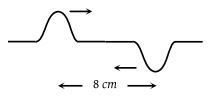1. Zero

2. Purely kinetic

3. Purely potential

4. Partly kinetic and partly potential

Concept Questions :-

Energy of waves
High Yielding Test Series + Question Bank - NEET 2020

Difficulty Level:

The diagram below shows the propagation of a wave. Which points are in the same phase : [AIIMS 1982]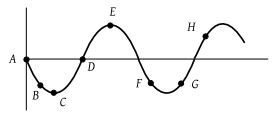(1) F, G

(2) C and E

(3) B and G

(4) B and F

Concept Questions :-

Wave motion
High Yielding Test Series + Question Bank - NEET 2020

Difficulty Level:

The correct graph between the frequency n and square root of density (ρ) of wire, keeping its length, radius and tension constant, is :

(1)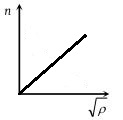(2)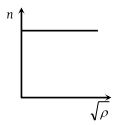(3)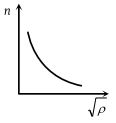(4)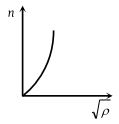Concept Questions :-

pressure wave in sound
High Yielding Test Series + Question Bank - NEET 2020

Difficulty Level:

If the speed of the wave shown in the figure is 330m/s in the given medium, then the equation of the wave propagating in the positive x-direction will be (all quantities are in M.K.S. units) :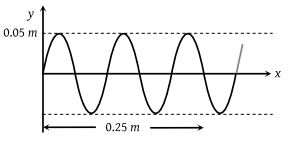(1) $y=0.05\mathrm{sin}2\pi \left(4000\text{\hspace{0.17em}}t-12.5\text{\hspace{0.17em}}x\right)$

(2) $y=0.05\mathrm{sin}2\pi \left(4000\text{\hspace{0.17em}}t-122.5\text{\hspace{0.17em}}x\right)$

(3) $y=0.05\mathrm{sin}2\pi \left(3300\text{\hspace{0.17em}}t-10\text{\hspace{0.17em}}x\right)$

(4) $y=0.05\mathrm{sin}2\pi \left(3300\text{\hspace{0.17em}}x-10\text{\hspace{0.17em}}t\right)$

Concept Questions :-

Wave motion
High Yielding Test Series + Question Bank - NEET 2020

Difficulty Level:

The displacement-time graphs for two sound waves A and B are shown in the figure, then the ratio of their intensities IA/IB is equal to :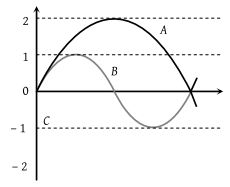1. 1 : 4

2. 1 : 16

3. 1 : 2

4. 1 : 1

Concept Questions :-

Energy of waves
High Yielding Test Series + Question Bank - NEET 2020

Difficulty Level:

A wave motion has the function $y={a}_{0}\mathrm{sin}\left(\omega \text{\hspace{0.17em}}t-kx\right)$. The graph in figure shows how the displacement y at a fixed point varies with time t. Which one of the labeled points shows a displacement equal to that at the position $x=\frac{\pi }{2k}$ at time t = 0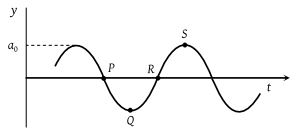(1) P

(2) Q

(3) R

(4) S

Concept Questions :-

Wave motion
High Yielding Test Series + Question Bank - NEET 2020

Difficulty Level:

Two loudspeakers L1 and L2 driven by a common oscillator and amplifier, are arranged as shown. The frequency of the oscillator is gradually increased from zero and the detector at D records a series of maxima and minima. If the speed of sound is 330 ms–1 then the frequency at which the first maximum is observed is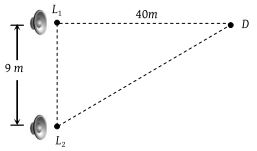(1) 165 Hz

(2) 330 Hz

(3) 496 Hz

(4) 660 Hz

Concept Questions :-

Doppler effect